# Class 9 RD Sharma Solutions – Chapter 8 Introduction to Lines and Angles- Exercise 8.2 | Set 2

• Last Updated : 16 May, 2022

### Question 11. In Figure, ACB is a line such that ∠DCA = 5x and ∠DCB = 4x.  Find the value of x.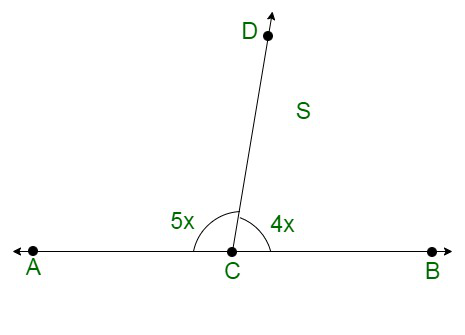Solution:

It is given that ACB is a line in the figure given below.

Thus, ∠ACD and ∠BCD form a linear pair.

Therefore, their sum must be equal to 180°.

Or we can say that

∠ACD + ∠BCD = 180°

Also, ∠ACD = 4x and ∠BCD = 5x.

This further simplifies to:

4x + 5x = 180

9x = 180

x =x = 20°

### Question 12. In the given figure, ∠POR = 3x and ∠QOR = 2x + 10, find the value of x for which POQ will be a line.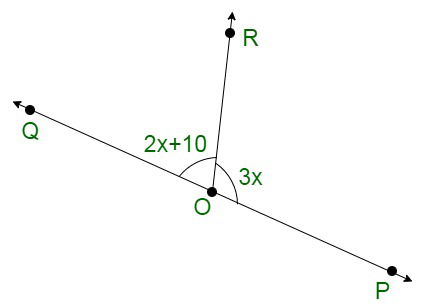Solution:

Here we have POQ as a line

So, ∠POR and ∠QOR form a linear pair.

Therefore, their sum must be equal to 180°

Or

∠POR + ∠QOR = 180°

It is given that ∠POR = (3x)° and ∠QOR = (2x + 10)°. On substituting these values above we get,

3x + (2x + 10) = 180°

3x + 2x + 10 = 180°

5x + 10 = 180°

5x = 180 – 10

5x = 170

x =x = 34°

### Question 13. In the given figure, a is greater than b by one-third of a right-angle. Find the values of a and b.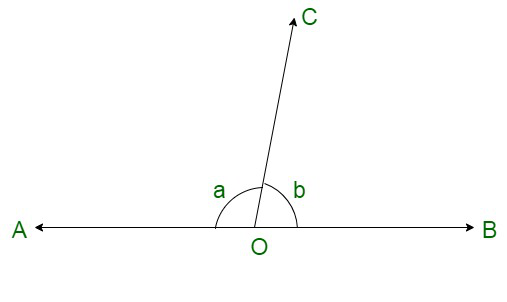Solution:

It is given that in the figure given below; a is greater than b by one-third of a right angle.

Or we can say that, the difference between a and b isi.e.

a – b =a – b = 30° ……..(i)

Also a and b forn a linear pair. Therefore, their sum must be equal to 180°.

We can say that:

a + b = 180° ……….(ii)

On adding (i) and (ii), we get:

2a = 180 + 30

2a = 210

a =a = 105°

On putting a = 105 in (i)

105 – b = 30

-b = 30 – 105

-b = -75

b = 75°

Hence, a = 105° and b = 75°

### Question 14. What value of y would make AOB a line in the given figure, if ∠AOC = 4y and ∠BOC = (6y + 30)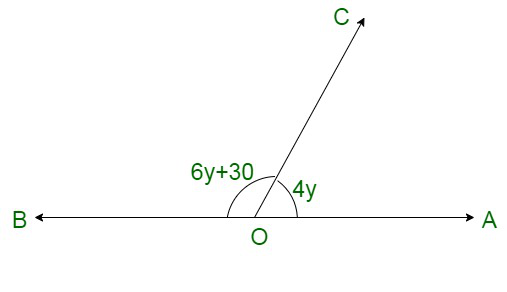Solution:

Let us assume, AOB as a straight line.

This makes ∠AOC and ∠BOC  to form a linear pair. Therefore, their sum must be equal to 180°.

We can say that:

∠AOC + ∠BOC = 180°

Also, ∠AOC = 4y  and ∠BOC = 6y + 30. This further simplifies to:

4y + (6y + 30) = 180

10y + 30 = 180

10y = 180 – 30

10y = 150

y =y = 15°

Thus, the value of y = 15° make AOB as a line.

### Question 15. If the given figure, ∠AOF and ∠FOG form a linear pair.

∠EOB = ∠FOC = 90° and ∠DOC = ∠FOG = ∠AOB = 30°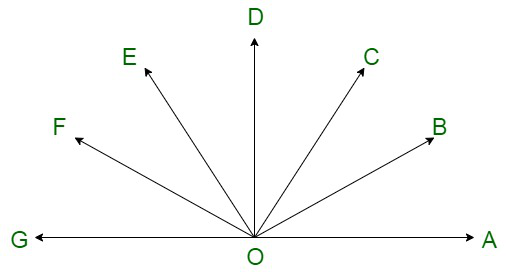(i) Find the measure of ∠FOE, ∠COB and ∠DOE.

(ii) Name all the right angles.

(iii) Name three pairs of adjacent complementary angles.

(iv) Name three pairs of adjacent supplementary angles.

(v) Name three pairs of adjacent angles.

Solution:

The given figure is as follows:

(i) It is given that ∠AOB, ∠FOE, ∠EOB and ∠FOG form a linear pair.

Therefore, their sum must be equal to 180°

i.e.

∠AOB + ∠FOE + ∠EOB + ∠FOG = 180°

It is given that:

∠FOG = 30°

∠AOB = 30°

∠EOB = 90° in equation above, we get:

∠AOB + ∠FOE + ∠EOB + ∠FOG = 180°

30° + ∠FOE + 90° + 30° = 180°

∠FOE + 150° = 180°

∠FOE = 180° – 150°

∠FOE = 30°

It is given that

∠FOC = 90°

From the above figure:

∠FOE + ∠DOE + ∠COD = 90°

30° + ∠DOE + 30° = 90°

∠DOE + 60° = 90°

∠DOE = 90° – 60°

∠DOE = 30°

Similarly, we have:

∠EOB = 90°

From the above figure:

∠DOE + ∠DOC + ∠COB = 90°

30° + 30° + ∠COB = 90°

∠COB + 60° = 90°

∠COB = 90° – 60°

∠COB = 30°

(ii) We have:

∠FOG = 30°

∠FOE = 30°

∠EOD = 30°

∠COD = 30°

∠COB = 30°

∠AOB = 30°

From the figure above and the measurements of the calculated angles we get two right angles as ∠DOG and ∠AOD.

Two right angles are already given as ∠FOC and ∠EOB

(iii) We have to find the three pair of adjacent complementary angles.

We know that ∠EOB is a right angle.

Therefore,

∠EOC and ∠COB are complementary angles.

Similarly, ∠AOD is a right angle.

Therefore,

∠AOC and ∠COD are complementary angles.

(iv) We have to find the three pair of adjacent supplementary angles.

Since, ∠AOG is a straight line.

Therefore, following are the three linear pair, which are supplementary:

∠AOB and ∠BOG

∠AOC and ∠COG

∠AOD and ∠DOG

(v) We have to find pair of adjacent angles, which are as follows:

∠AOB and ∠BOC

∠COD and ∠DOE

∠EOF and ∠FOG

### Question 16. In the given figure, OP, OQ , OR, and OS are four rays. Prove that:

∠POQ + ∠QOR + ∠SOR + ∠POS = 360°.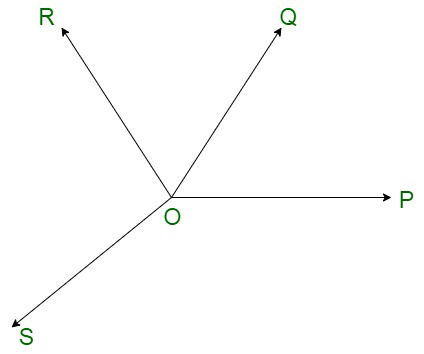Solution:

Let us draw TOP as a straight line.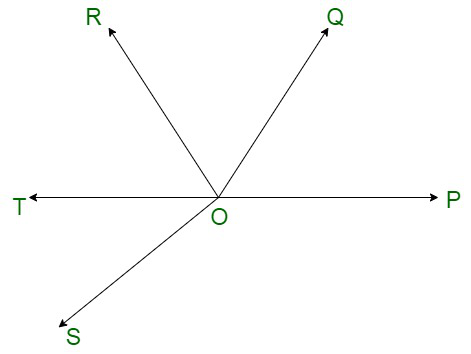Since, TOP is a line, therefore, ∠POQ, ∠QOR and ∠ROT form a linear pair.

Also, ∠POS and ∠SOT form a linear pair.

Thus, we have:

∠POQ + ∠QOR + ∠ROT = 180° ……(i)

and

∠POS + ∠SOT = 180°  …….(ii)

On adding (i) and (ii), we get;

(∠POQ + ∠QOR + ∠ROT) + (∠POS + ∠SOT) = 180° + 180°

∠POQ + ∠QOR + (∠ROT + ∠SOT) + ∠POS = 360°

∠POQ + ∠QOR + ∠SOR + ∠POS = 360°

Hence proved.

### Question 17. In the given figure, ray OS stand on a line POQ, Ray OR and ray OT are angle bisectors of ∠POS and∠SOQ respectively. If ∠POS = x, find ∠ROT.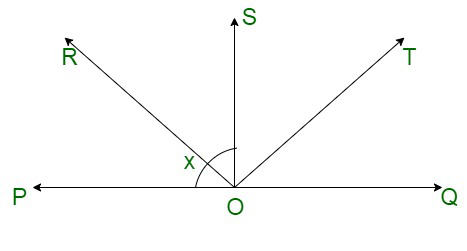Solution:

In the figure given below, we have

Ray OR as the bisector of ∠POS

Therefore,

∠POR = ∠ROS

or,

∠POS = 2∠ROS ………..(i)

Similarly, ray OT as the bisector of ∠SOQ

Therefore,

∠TOQ = ∠TOS

or,

∠QOS = 2∠TOS ……….(ii)

Also, Ray OS stand on a line POQ. Therefore, ∠POS and ∠QOS form a linear pair.

Thus,

∠POS + ∠QOS = 180°

From (i) and (ii)

2∠ROS + 2∠TOS = 180°

2(∠ROS + ∠TOS) = 180°

∠ROS + ∠TOS =∠ROT = 90°

### Question 18. In the given figure, lines PQ and RS intersect each other at point O. If ∠POR: ∠ROQ = 5:7, find all the angles.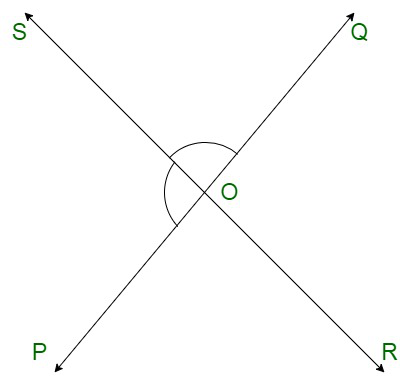Solution:

Let ∠POR and ∠ROQ be 5x and 7x respectively.

Since, Ray OR stand on line POQ. Thus, ∠POR and ∠ROQ form a linear pair.

Therefore, their sum must be equal to 180°.

Or,

∠POR + ∠ROQ = 180°

5x + 7x = 180°

12x = 180°

x =x = 15° …….(i)

Thus,

∠POR = 5x

= 5(15)

= 75

∠POR = 75°

Thus,

∠ROQ = 7x

= 7(15)

=105

∠ROQ = 105°

It is evident from the figure, that ∠QOS and ∠POR are vertically opposite angles.

And we know that vertically opposite angles are equal.

Therefore,

∠QOS = ∠POR

∠QOS = 75°

Similarly, ∠POS and ∠ROQ are vertically opposite angles.

And we know that vertically opposite angles are equal.

Therefore,

∠POS = ∠ROQ

∠POS = 105°

### Question 19. In the given figure, POQ is a line. Ray OR is perpendicular to line PQ. OS is another ray lying between rays OP and OR. Prove that

∠ROS = 1212 (∠QOS − POS).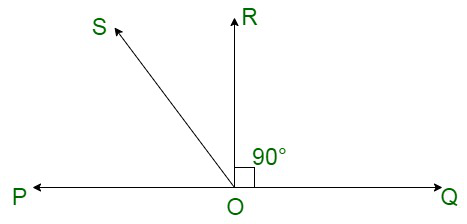Solution:

The given figure shows:

We have POQ as a line. Ray OR is perpendicular to line PQ. Therefore,

∠ROQ = 90°

∠POR = 90°

From the figure above, we get

∠ROS + ∠POS = 90° ………(i)

∠POS and ∠QOS form a linear pair.

Therefore,

∠QOS + ∠POS = 180°   ……(ii)

From (i) and (ii) equation we get:

∠QOS + ∠POS = 2 × 90 ∠QOS + ∠POS = 2 × 90

∠QOS + ∠POS = 2(∠ROS + ∠POS)

2∠ROS = ∠QOS – ∠POS

∠ROS =(∠QOS – ∠POS)

Hence, proved.

My Personal Notes arrow_drop_up